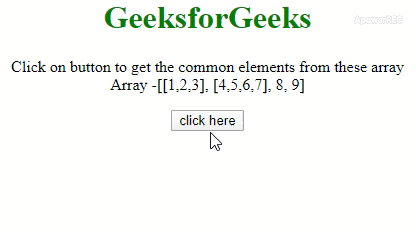# How to flatten array with the JavaScript/jQuery ?

JavaScript contains many arrays (or 2-d array) and the task is to flatten the array and make that look like 1-d JavaScript array. There are two approaches that are discussed below. You can also use Underscore.js _.flatten() with Examples.

Approach 1: Use Array.prototype.concat.apply() method to perform the operation. The concat() and apply() method is used to concat the arrays to 1-d array.

• Example:

 ` ` `<``html``> ` ` `  `<``head``> ` `    ``<``title``> ` `        ``How to flatten array with ` `        ``the JavaScript? ` `    `` ` `     `  `    ``<``style``> ` `        ``body { ` `            ``text-align: center; ` `        ``} ` `         `  `        ``h1 { ` `            ``color: green; ` `        ``} ` `    `` ` ` ` ` `  `<``body``> ` `    ``<``h1``>GeeksforGeeks ` `     `  `    ``<``p` `id``=``"GFG_UP"``> ` `     `  `    ``<``button` `onClick``=``"GFG_Fun()"``> ` `        ``click here ` `    `` ` `     `  `    ``<``p` `id``=``"GFG_DOWN"``> ` `     `  `    ``<``script``> ` `        ``var up = document.getElementById('GFG_UP'); ` `        ``var down = document.getElementById('GFG_DOWN'); ` `        ``var arr1 = [1, 2, 3]; ` `        ``var arr2 = [4, 5, 6, 7]; ` `        ``var arr = [arr1, arr2, 8, 9]; ` `         `  `        ``up.innerHTML = "Click on button to get " ` `                ``+ "the common elements from these" ` `                ``+ " array <``br``>Array -[[" + arr ` `                ``+ "], [" + arr + "], " + arr ` `                ``+ ", " + arr + "]"; ` ` `  `        ``function GFG_Fun() { ` `            ``down.innerHTML =  ` `                ``Array.prototype.concat.apply([], arr); ` `        ``} ` `    `` ` ` ` ` `  ` `

• Output:Approach 2: The \$.map() method in jQuery can be used to perform the operation. This method takes the array and a method as input. The second argument which is a method takes elements of original array one by one and return its elements.

• Example:

 ` ` `<``html``> ` ` `  `<``head``> ` `    ``<``title``> ` `        ``How to flatten array with ` `        ``the JavaScript? ` `    `` ` `     `  `    ``<``style``> ` `        ``body { ` `            ``text-align: center; ` `        ``} ` `         `  `        ``h1 { ` `            ``color: green; ` `        ``} ` `    `` ` `    ``<``script` `src``= ` `"https://ajax.googleapis.com/ajax/libs/jquery/3.4.0/jquery.min.js"``> ` `    `` ` ` ` ` `  `<``body``> ` `    ``<``h1``>GeeksforGeeks ` `     `  `    ``<``p` `id``=``"GFG_UP"``> ` `     `  `    ``<``button` `onClick``=``"GFG_Fun()"``> ` `        ``click here ` `    `` ` `     `  `    ``<``p` `id``=``"GFG_DOWN"``> ` `     `  `    ``<``script``> ` `        ``var up = document.getElementById('GFG_UP'); ` `        ``var down = document.getElementById('GFG_DOWN'); ` `        ``var arr1 = [1, 2, 3]; ` `        ``var arr2 = [4, 5, 6, 7]; ` `        ``var arr = [arr1, arr2, 8, 9]; ` `         `  `        ``up.innerHTML = "Click on button to get the" ` `                ``+ " common elements from these array" ` `                ``+ "<``br``>Array - [[" + arr + "], [" ` `                ``+ arr + "], " + arr + ", "  ` `                ``+ arr + "]"; ` ` `  `        ``function GFG_Fun() { ` `            ``down.innerHTML = \$.map(arr, function(n) { ` `                ``return n; ` `            ``}); ` `        ``} ` `    `` ` ` ` ` `  ` `

• Output:My Personal Notes arrow_drop_upCheck out this Author's contributed articles.

If you like GeeksforGeeks and would like to contribute, you can also write an article using contribute.geeksforgeeks.org or mail your article to contribute@geeksforgeeks.org. See your article appearing on the GeeksforGeeks main page and help other Geeks.

Please Improve this article if you find anything incorrect by clicking on the "Improve Article" button below.

Be the First to upvote.

Please write to us at contribute@geeksforgeeks.org to report any issue with the above content.